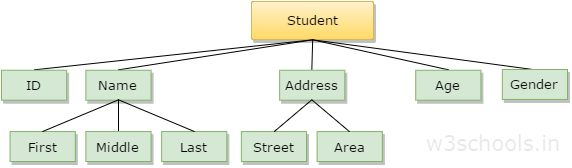## Data Structure Tutorial Index

In the modern world, data and its information have significance, and different implementations take place to store it in different ways. Data is simply a collection of facts and figures, or you can say that data is a set of values or values in a particular format that refers to a single set of item values. The data items are then classified into sub-items, the group of items that are not called the simple primary form of the item.

Let's take an example where a student's name can be broken down into three sub-items: first, middle, and last. But, an ID assigned to a student will usually be considered a single item.The example mentioned above, such as ID, Age, Gender, First, Middle, Last, Street, Area, etc., are elementary data items, whereas the Name and the Address are group data items.

## What is Data Structure?

In the context of computers, the data structure is a specific way of storing and organizing data in the computer's memory so that these data can be easily retrieved and efficiently used when needed later. The data can be managed in many ways, such as a logical or mathematical model for a particular data organization called a data structure.

The variety of a specific data model depends on two factors:

• First, it must be loaded enough into the structure to reflect the actual relationship of the data with a real-world object.
• Second, the formation should be so simple that one can efficiently process the data whenever necessary.

## Categories of Data Structure

Data structures can be subdivided into two major types:

• Linear Data Structure
• Non-linear Data Structure

### Linear Data Structure

A data structure is said to be linear if its elements combine to form any specific order. There are two techniques for representing such linear structure within memory.

• The first way is to provide a linear relationship between all the elements represented using a linear memory location. These linear structures are called arrays.
• The second technique provides a linear relationship between all the elements represented using the concept of pointers or links. These linear structures are called linked lists.

The typical examples of the linear data structure are:

• Arrays
• Queues
• Stacks

### Non-linear Data Structure

This structure mainly represents data with a hierarchical relationship between different elements.

Examples of Non-Linear Data Structures are listed below:

• Graphs
• Family of trees and